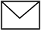# Common Nails, BWG21 Galvanized Wires for Yemen Client

Our common nail & BWG21 galvanized wires to Yemen, with full details like the following.

1. First container 20 ft., common nails, carton 16/kg and 16kg/boxes and one box 1kg.
1. (1.5 × 12) quantity is (200/cartons).
2. (2 × 11) quantity is (1000/cartons).
3. (2.5 × 11) quantity is (100/cartons).
4. (3 × 10) quantity is (100/cartons).
5. (4 × 8) quantity is (100/cartons).
6. (5 × 6) quantity is (50/cartons).
7. (6 × 6) quantity is (100/cartons).
2. Second container 20 ft., common nails, carton 16/kg and 16/boxes and one box 1/kg.
1. (1.5 × 14) quantity is (250/cartons).
2. (1.5 × 12) quantity is (150/cartons).
3. (2 × 11) quantity is (400/cartons).
4. (2.5 × 11) quantity is (150/cartons).
5. (3 × 10) quantity is (100/cartons).
6. (4 × 8) quantity is (300/cartons).
7. (5 × 6) quantity is (100/cartons).
8. (6 × 6) quantity is (100/cartons).
3. Third container 20ft, bwg21 galvanized wire, roll weight 3/kg, bundle coil 5/rolls, quantity (25.6/ton).

Inquiry for Our Product

Anping County Wennian Wire Mesh Products Co., Ltd.
E-mail: sales@wnmesh.com